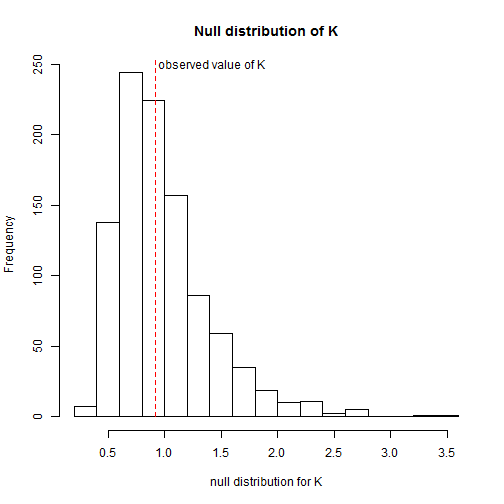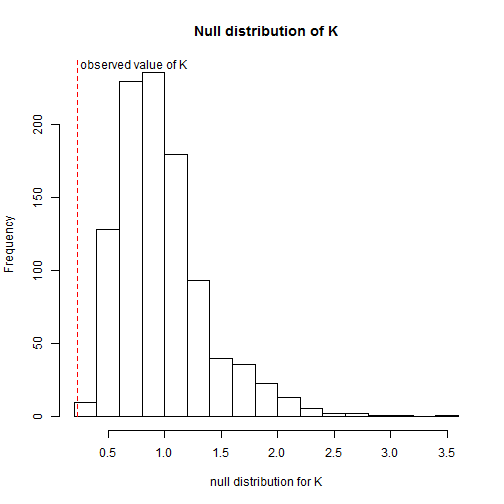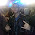## Tuesday, December 2, 2014

### Testing a hypothesis that phylogenetic signal is different from 1.0

“A reviewer asked us to test if phylogenetic signal is equal to 1, but I have not found any package that does that. Do you have any suggestion about how to do this?

Since phylogenetic signal (measured using either Pagel's λ or Blomberg's K) has an expected value of 1.0 under Brownian motion; testing whether signal is different from 1.0 is essentially testing whether phylogenetic signal is smaller or greater than if the data arose by a Brownian process.

We can do this with a likelihood-ratio test (with some caveats) if we are using Pagel's λ to measure phylogenetic signal. If we are using Blomberg's K then we can use simulation (with fewer caveats).

Here's a quick demo.

First, let's simulate some data with & without signal. For the no-signal data I will just randomly permute the Brownian data:

``````library(phytools)
## tree
tree<-pbtree(n=26,tip.label=LETTERS)
## data with signal
x1<-fastBM(tree)
## data without signal
x2<-setNames(sample(x1),names(x1))
``````

Now let's estimate Pagel's lambda for both datasets. In each case, we will compare the likelihood of the fitted model to the likelihood of λ = 1.

``````## Pagel's lambda
## first the data with signal
lam1<-phylosig(tree,x1,method="lambda")
lam1
``````
``````## \$lambda
##  0.9413
##
## \$logL
##  -38.39
``````
``````fitBrownian<-brownie.lite(paintSubTree(tree,tree\$edge[1,1],state="1"),x1)
fitBrownian
``````
``````## ML single-rate model:
##  s^2 se  a   k   logL
## value    1.1035  0.3061  -0.2291 2   -38.6817
##
## ML multi-rate model:
##  s^2(1)  se(1)   a   k   logL
## value    1.1035      -0.2291 2   -38.6817
##
## P-value (based on X^2): 1
##
## R thinks it has found the ML solution.
``````
``````## likelihood ratio test
LR<-2*(lam1\$logL-fitBrownian\$logL1)
LR
``````
``````##  0.5774
``````
``````P.lr<-pchisq(LR,df=1,lower.tail=FALSE)
P.lr
``````
``````##  0.4473
``````
``````## now the data without signal
lam2<-phylosig(tree,x2,method="lambda")
lam2
``````
``````## \$lambda
##  6.917e-05
##
## \$logL
##  -46.33
``````
``````fitBrownian<-brownie.lite(paintSubTree(tree,tree\$edge[1,1],state="1"),x2)
fitBrownian
``````
``````## ML single-rate model:
##  s^2 se  a   k   logL
## value    4.5548  1.2633  0.0452  2   -57.112
##
## ML multi-rate model:
##  s^2(1)  se(1)   a   k   logL
## value    4.5548  1   0.0452  2   -57.112
##
## P-value (based on X^2): 1
##
## Optimization may not have converged.
``````
``````## likelihood ratio test
LR<-2*(lam2\$logL-fitBrownian\$logL1)
LR
``````
``````##  21.56
``````
``````P.lr<-pchisq(LR,df=1,lower.tail=FALSE)
P.lr
``````
``````##  3.428e-06
``````

Now let's do roughly the same thing for Blomberg's K. In this case, however, we will use simulation by Brownian motion to get a null distribution for K.

``````## Blomberg's K
## first the data with signal
K1<-phylosig(tree,x1)
K1
``````
``````##  0.9221
``````
``````## fit a Brownian model to the data to get parameters
## for simulation
fitBrownian<-brownie.lite(paintSubTree(tree,tree\$edge[1,1],state="1"),x1)
X<-fastBM(tree,sig2=fitBrownian\$sig2.single,a=fitBrownian\$a.single,nsim=999)
nullK<-apply(X,2,phylosig,tree=tree)
mean(nullK)
``````
``````##  0.9857
``````
``````hist(nullK,breaks=20,xlab="null distribution for K",
main="Null distribution of K")
lines(c(K1,K1),c(0,par()\$usr),lty="dashed",col="red")
text(x=K1,y=0.985*par()\$usr,"observed value of K",pos=4,offset=0.2)
``````The distribution is of K under the null hypothesis is highly assymetric, so it might make sense to conduct a test of K using `abs(log(K))`. (Just an idea.)

``````P.k<-mean(abs(log(c(K1,nullK)))>=abs(log(K1)))
P.k
``````
``````##  0.849
``````

Now let's do the same with the no-signal data:

``````K2<-phylosig(tree,x2)
K2
``````
``````##  0.2261
``````
``````## fit a Brownian model to the data to get parameters
## for simulation
fitBrownian<-brownie.lite(paintSubTree(tree,tree\$edge[1,1],state="1"),x2)
X<-fastBM(tree,sig2=fitBrownian\$sig2.single,a=fitBrownian\$a.single,nsim=999)
nullK<-apply(X,2,phylosig,tree=tree)
mean(nullK)
``````
``````##  0.9844
``````
``````hist(nullK,breaks=20,xlab="null distribution for K",
main="Null distribution of K")
lines(c(K2,K2),c(0,par()\$usr),lty="dashed",col="red")
text(x=K2,y=0.985*par()\$usr,"observed value of K",pos=4,offset=0.2)
````````````P.k<-mean(abs(log(c(K2,nullK)))>=abs(log(K2)))
P.k
``````
``````##  0.001
``````

That's it.

1.Note that a couple of caveats apply - particularly to testing hypothesis about λ using the likelihood ratio:

(1) The likelihood-ratio test, in which we use a Χ^2distribution for hypothesis testing, depends on asymptotic properties of likelihoods which do not hold for small sample sizes (and small trees).

(2) There is also a problem when we are at the bounds of a particular parameter - and this will be true near 0.0 & 1.0 for λ.

For more details, see Carl Boettiger's paper which I believe can be found here.

1.This comment has been removed by the author.

2.thanks Liam. I was able to run this for most variables but in some cases I got this error when applying the brownie.lite function:

Error in solve.default(model1\$hessian) :
Lapack routine dgesv: system is exactly singular: U[2,2] = 0

Do you know what could be the problem?

Marcelo

1.Hola Marcelo.

Since this looks like it is trying to invert the Hessian matrix, it may just be involved in computing standard errors for the parameter estimates (and not the optimization). Did the function still return an optimized λ in this case.

Saludos, Liam

3.Nope, it did not. Thanks Liam!

4.Hello Mr. Revell,

I work with Ecology and Evolution in Tropical Forests and I'm still few familiar with Pagel's Lambda and the function provided by "phytools" to calculate it and I have a doubt in some results I got here.

For instance, in one of results I got: Lamba =1.00033; logL
= -769.7819; logL0 = -1313.418 and P-value of pchisq to the likelihood test = 1.928349e-238.

In principle, I thought these values a bit wierd. So, I checked everything In my phylo tree and trait matrix and apparentely everything was correct, even because I perfectly ran the phylo signal test using Blomberg's K.

Thus, my doubt is centered exactly in the fact Lambda and p value above. Lamba is subtly > 1 and p - value is signiticant. In my opinion the lambda is quite close to entire 1 and p value very high. I mean, lambda seems more consistent with Brownian Motion (Lambda = 1) and p-value is significant. Perhaps, it's a stupid misleading I'm doing, but one lamba so close to one like should present significant p-value in the likelihood test?

I read some publications to better interpret this result, but I remained with this doubt: could I consider this result like significant phylo signal or more congruent with Brownian Motion?

Thank you very much,

Écio

5.Hi Liam and phytools users,

I have a question about the interpretation of phylogenetic signal (specifically, pagel's lambda). I calculated the phylogenetic signal over multiple trees and for each tree I obtained the value of lambda and to evaluate if lambda is significantly different from 0 (with test=TRUE in phylosig). Additionally I performed a likelihood ratio test to evaluate in each case if the phylogenetic signal is different from 1 (as in this post).

Overall I got that the phylogenetic signal over all trees has a mean of 0.89 (0.8-0.96) and is always significantly different from 0. However, the p-value when comparing 1 is sometimes much lower than 0.05 and sometimes higher. Would you still consider this as a 'high' phylogenetic signal even if deviates from 1 in some cases? I appreciate all your opinions and insight!

Thank you all very much for your time and help!

Best,

Laura

Note: due to the very large amount of spam, all comments are now automatically submitted for moderation.